# Propulsion and GNC¶

#### V. Hunter Adams (vha3), MAE 4160/5160, Spring 2020¶

In :
from IPython.display import Latex
from IPython.display import Image
from IPython.core.display import HTML


## Orbital Maneuvers¶

### Vis-Viva Equation¶

In the absence of external forces, like drag, the total energy of a spacecraft in orbit (kinetic + potential) is conserved. This conservation of energy, coupled with conservation of angular momentum, can be used to derive the vis viva equation shown below.

\begin{align} \frac{v^2}{2} - \frac{GM}{r} &= -\frac{GM}{2a} = \epsilon \end{align}

where:

\begin{align} v&: \text{ spacecraft velocity}\\ G&: \text{Newton's gravitational constant}\\ M&: \text{Mass of planet}\\ r &: \text{ distance separating the two bodies}\\ a &: \text{ semi-major axis ($a > 0$ for ellipses, $a=\infty$ for parabolas, $a<0$ for hyperbolas)} \end{align}

For a full derivation of the above equation, see Wikipedia. The vis viva equation is tremendously useful for performing $\Delta v$ calculations for in-plane orbital maneuvers like Hohmann Tranfers, as we'll discuss momentarily. It is also useful for calculating escape velocity, as shown below.

Example Question: Find the expression for the escape velocity from the surface of a planet with radius $r_p$ and mass $M$.

Example Solution: Begin by rearranging the vis viva equation to solve for velocity:

\begin{align} v^2 &= GM\left(\frac{2}{r} - \frac{1}{a}\right) \end{align}

To escape the planet, we want to achieve an orbit with a perigee altitude equal to the radius of that planet, $r_p$, and a semi-major axis of $\infty$. So, substitute into the above equation:

\begin{align} v^2 &= GM \left(\frac{2}{r_p} - \frac{1}{\infty}\right)\\ &= GM\left(\frac{2}{r_p} - 0\right)\\ &= \frac{2GM}{r_p} \end{align}

Thus:

\begin{align} \boxed{v_{esc} = \sqrt{\frac{2GM}{r_p}}} \end{align}

### In-Plane Maneuvers¶

The following analysis assumes impulsive maneuvers. We assume that the burn time for the thruster is very short compared to the orbital period ($\Delta t_b << T$), and we assume that the spacecraft's change in position during the burn is negligible. Under these assumptions, the new orbit can be found from the current position ($\textbf{r}_{new} = \textbf{r}_{old}$), and the new velocity can be found as shown below:

\begin{align} \textbf{V}_{new} &= \textbf{V}_{old} + \Delta \textbf{V} \end{align}

and where $\Delta \textbf{V}$ can be found by finding the instantaneous change in momentum, as shown below:

\begin{align} \textbf{F} &= \frac{d}{dt}\left(m \textbf{V}\right) = m \frac{d}{dt} \textbf{V} = m \frac{\Delta \textbf{V}}{\Delta t} \longrightarrow \Delta \textbf{V} = \frac{\textbf{F}}{m}\Delta t \end{align}

The consequence of these assumptions is that a spacecraft in an elliptical orbit will always return to the location at which the last maneuver was made. For chemical propulsion (characterized by high thrust and short burns), these are good approximations. For electric propulsion (low thrust and long burns), they are not.

Under these assumptions, the spacecraft will instantaneously move from one orbit to another orbit during a maneuver. So, we calculate the required $\Delta \textbf{V}$ for that maneuver as:

\begin{align} \Delta\textbf{V} &= \textbf{V}_{target} - \textbf{V}_{current} \end{align}

The velocity for the target orbit, minus the velocity for the orbit that you're currently on. Let's look at some examples.

#### Hohmann Transfer¶

Typically, an in-plane maneuver is performed in order to raise the semimajor axis $a$. However, it can also be used to change the eccentricity $e$ or the argument of periapsis $\omega$. For maximum efficiency (least amount of propellant required), these burns should be performed tangent to the orbit.

The Hohmann Transfer is one of the most common in-plane maneuvers. It is a two-burn maneuver for moving from one circular orbit to another circular orbit in the same orbital plane.

We assume that we are on a circular orbit of radius $r_1$, and we would like to maneuver to another circular orbit with radius $r_2>r_1$, as shown below. The first burn will move us from our circular orbit to an elliptical transfer orbit with an apopapsis altitude equal to the radius of our goal circular orbit. The second burn, performed at apoapsis, raises the periapsis of the orbit to be equal to the apoapsis altitude (i.e. circularizes). Let's walk through this maneuver step by step.

In :
Image("one.png", width=300)

Out: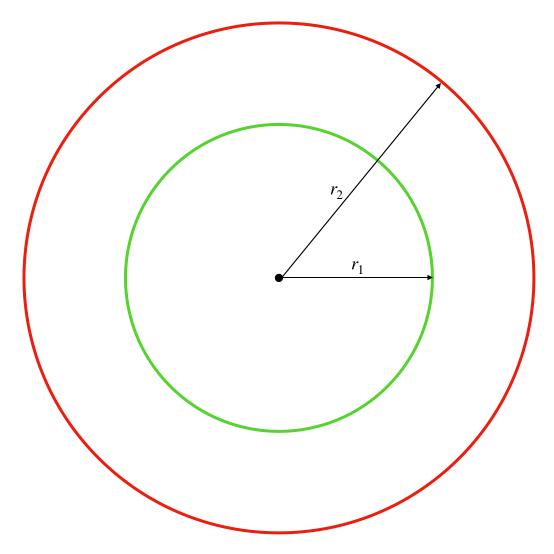1: Calculate the velocity of the spacecraft on the initial circular orbit using vis viva.

\begin{align} v_{c1} &= \sqrt{GM\left(\frac{2}{r} - \frac{1}{a}\right)}\\ &= \sqrt{GM\left(\frac{2}{r_1} - \frac{1}{r_1}\right)}\\ &= \sqrt{\frac{GM}{r_1}} \end{align}
In :
Image("two.png", width=300)

Out: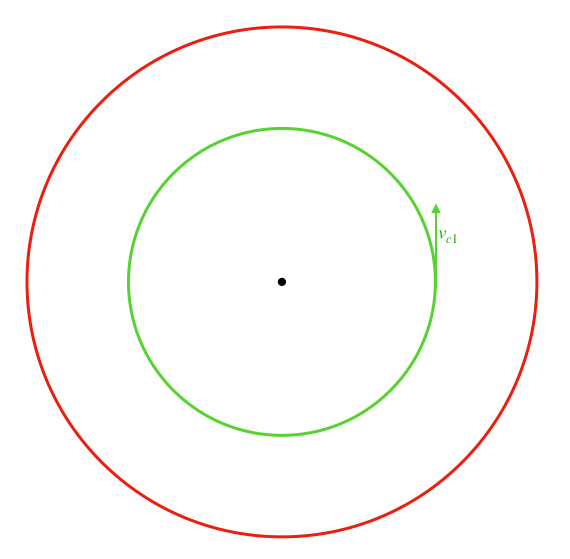2: Calculate the elliptical transfer orbit semimajor axis and eccentricity.

\begin{align} a_{T} &= \frac{r_1 + r_2}{2}\\ e_{T} &= \frac{r_1 - r_2}{r_1+r_2} \end{align}

3: Calculate the perigee velocity of the transfer orbit using vis viva.

\begin{align} v_{pT} &= \sqrt{GM\left(\frac{2}{r} - \frac{1}{a}\right)}\\ &= \sqrt{GM\left(\frac{2}{r_1} - \frac{1}{a_T}\right)}\\ &= \sqrt{GM\left(\frac{2}{r_1} - \frac{2}{r_1+r_2}\right)}\\ &= \sqrt{\frac{2GM}{r_1}\left(1 - \frac{1}{1+\frac{r_2}{r_1}}\right)}\\ &= \sqrt{\frac{GM}{r_1}}\sqrt{2\left(1 - \frac{1}{1+\frac{r_2}{r_1}}\right)}\\ \end{align}
In :
Image("three.png", width=300)

Out: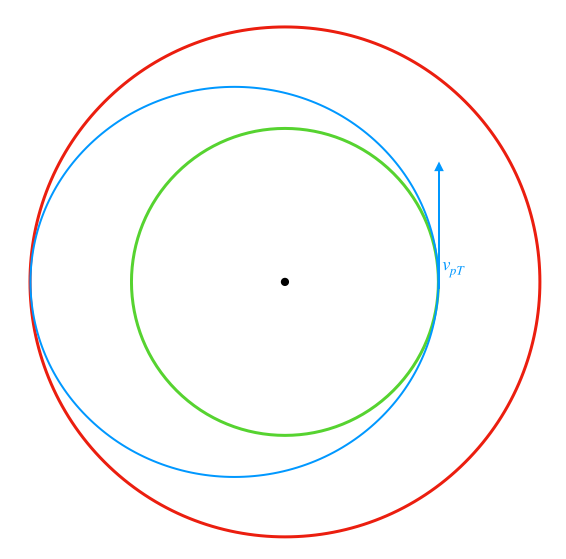4: Calculate the $\Delta V$ required for the first maneuver.

\begin{align} \Delta V_1 &= v_{pT} - v_{c1}\\ &= \sqrt{\frac{GM}{r_1}}\sqrt{2\left(1 - \frac{1}{1+\frac{r_2}{r_1}}\right)} - \sqrt{\frac{GM}{r_1}}\\ &= \sqrt{\frac{GM}{r_1}}\left[\sqrt{2\left(1 - \frac{1}{1+\frac{r_2}{r_1}}\right)} - 1\right] \end{align}

5: Calculate apogee velocity of transfer orbit using vis viva.

\begin{align} v_{aT} &= \sqrt{GM\left(\frac{2}{r} - \frac{1}{a}\right)}\\ &= \sqrt{GM\left(\frac{2}{r_2} - \frac{1}{a_T}\right)}\\ &= \sqrt{GM\left(\frac{2}{r_2} - \frac{2}{r_1+r_2}\right)}\\ &= \sqrt{\frac{2GM}{r_2}\left(1 - \frac{1}{1+\frac{r_1}{r_2}}\right)}\\ &= \sqrt{\frac{GM}{r_2}}\sqrt{2\left(1 - \frac{1}{1+\frac{r_1}{r_2}}\right)}\\ \end{align}
In :
Image("four.png", width=300)

Out: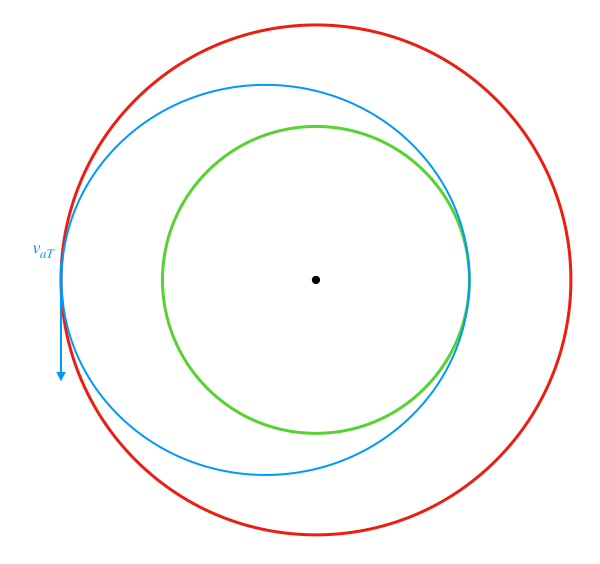6: Calculate the circular velocity of the final orbit using vis viva.

\begin{align} v_{c2} &= \sqrt{GM\left(\frac{2}{r} - \frac{1}{a}\right)}\\ &= \sqrt{GM\left(\frac{2}{r_2} - \frac{1}{r_2}\right)}\\ &= \sqrt{\frac{GM}{r_2}} \end{align}
In :
Image("five.png", width=300)

Out: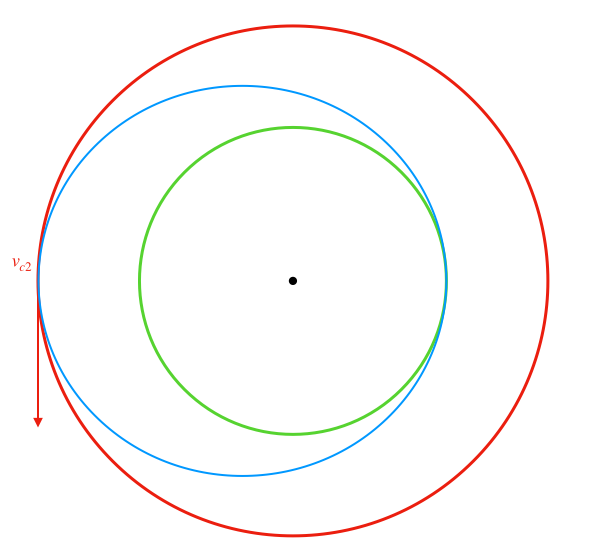7: Calculate the $\Delta V$ required for the second maneuver.

\begin{align} \Delta V_2 &= v_{c2} - v_{aT}\\ &= \sqrt{\frac{GM}{r_2}}\sqrt{2\left(1 - \frac{1}{1+\frac{r_1}{r_2}}\right)} - \sqrt{\frac{GM}{r_2}}\\ &= \sqrt{\frac{GM}{r_2}}\left[\sqrt{2\left(1 - \frac{1}{1+\frac{r_1}{r_2}}\right)} - 1\right] \end{align}

8: Calculate the total required $\Delta V$.

\begin{align} \Delta V_{tot} &= \Delta V_1 + \Delta V_2\\ &= \sqrt{\frac{GM}{r_1}}\left[\sqrt{2\left(1 - \frac{1}{1+\frac{r_2}{r_1}}\right)} - 1\right] + \sqrt{\frac{GM}{r_2}}\left[\sqrt{2\left(1 - \frac{1}{1+\frac{r_1}{r_2}}\right)} - 1\right] \end{align}

#### Rendezvous¶

Precisely the same analysis that we used for the above Hohmann Transfer is used for Rendezvous maneuvers, in which two spacecraft must come in close enough proximity to physically attach to one another. Typically, one spacecraft adjusts its orbit in order to intercept the target spacecraft. It will do so by performing a Hohmann Transfer from its initial parking orbit to an orbit that is nearly identical to the target spacecraft, but with slightly different semimajor axis $a$. This difference in semimajor axis gives the two spacecraft slightly different orbital periods, allowing them to catch up with one another. Once in proximity, the interceptor spacecraft will perform another burn to equalize semimajor axis with the target.

### Interplanetary Maneuvers¶

#### Spheres of influence¶

The vis viva equation above is for the two-body problem. For an interplanetary trajectory, there are more than two bodies involved and we must decide which body to use in our maneuver calculation. The sphere of influence of a body is the region around it in which it is the primary gravitational force on an orbiting object is that body. It tells us which central body to use for calculations at different points in the orbit. The sphere of influence is approximated by the equation below, the derivation of which can be found on Wikipedia and elsewhere:

\begin{align} r_{SOI} &= a\left(\frac{m}{M}\right)^{\frac{2}{5}} \end{align}

#### Hohmann Transfers¶

The general sequence for an interplanetary Hohmann Transfer is given below:

1. Circular orbit around departure planet
2. $\Delta V_1$
3. Hyperbolic orbit around departure planet/elliptical orbit around Sun
4. Elliptical orbit around Sun/hyperbolic orbit around destination planet
5. $\Delta V_2$
6. Circular orbit around destination planet

Where $\Delta V_1$ goes from $V$ at circular orbit around departure planet to $V$ at pericenter of hyperbola around departure planet with $V_{\infty} = \text{heliocentric velocity at departure position of transfer orbit - heliocentric velocity of departure planet}$.

And $\Delta V_2$ goes from $V$ at pericenter of hyperbola around target planet with $V_{\infty} = \text{heliocentric velocity of target planet - heliocentric velocity at target position of transfer orbit}$ to $V$ at circular orbit around target planet.

The spacecraft starts on a circular orbit around the departure planet. Through $\Delta V_1$, it puts itself on a hyperbolic orbit with respect to the departure planet and an elliptical orbit with respect to the Sun. This elliptical transfer orbit carries it to the vicinity of the destination planet. It has a hyperbolic orbit with respect to the destination planet. Through $\Delta V_2$, it places itself on a circular orbit with respect to the destination planet.

#### Flyby¶

Flyby maneuvers are an extremely common method for adding $\Delta V$ to a spacecraft, and changing its trajectory, without consuming any propellant. The geometery of a hyperbolic flyby is shown below.

In :
Image("fllyby.png", width=400)

Out: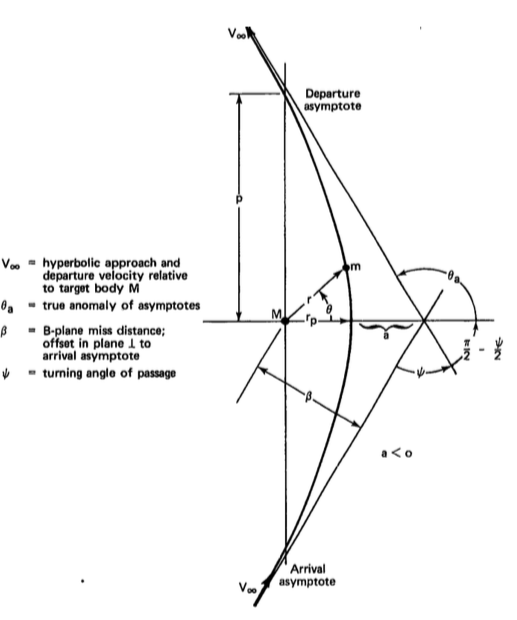The general principles for a flyby are given below:

1. Relative to the planet, the spacecraft's approach and departure velocities are the same.
2. The spacecraft departs the planet at a different angle than it approached the planet, however. This change in angle creates $\Delta V$ with respect to the Sun. The larger the planet, the larger the $\Delta V$.

The turning angle of passage can be calculated from the eccentricity, as shown below.

\begin{align} \sin{\frac{\Psi}{2}} &= \frac{1}{e} = \left(1 + \frac{V_{\infty}^2r_p}{\mu}\right)^{-1} \end{align}

And the $\Delta V$ with respect to the Sun can be calculated from this angle:

\begin{align} \Delta V &= 2V_{\infty} \sin\left(\frac{\Psi}{2}\right) \end{align}

### Out-of-plane maneuvers¶

In an out-of-plane maneuver, a component of the $\Delta V$ is perpendicular to the orbital plane. This is typically performed in order to change $i$ or $\Omega$, but it is costly. In general, you want to allow for physics to help you with your out-of-plane maneuvers in order to save propellant. We can use nodal precession, for example, to change $\Omega$. Let's consider the case where we'd like to perform a burn to change orbit inclination. In this case the magnitude of the velocity before and after the burn is the same, but the direction is different. See below.

In :
Image("pc.png", width=200)

Out: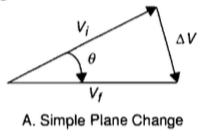The most efficient place to perform such a manuever is at the nodes (where the orbit crosses the equatorial plane). At the nodes the $\Delta V$ required in order to induce some change in inclination $\Delta i$ is given by:

\begin{align} \Delta V &= 2V_i \sin{\left(\frac{\Delta i}{2}\right)} \end{align}

You can see that this $\Delta V$ is proportional to $V_i$. These maneuvers cost a lot of propellant.

## Propulsion¶

We use propulsion to execute the manuevers described above. This section first discusses the Rocket Equation, which is the foundational equation for in-space propulsion, and then discusses various flavors of thrusters.

### Rocket Equation¶

Suppose a rocket which is ejecting fuel, as shown in the image below from Wikipedia. Our goal is to find an equation that relates the $\Delta V$ generated for the rocket in terms of the dry mass of the rocket, the propellant mass within the rocket, and the ejection velocity of the propellant.

In :
Image("rocket.png", width=800)

Out:The net force acting on the entire system (rocket + ejected mass) is given by the change in momentum of the entire system. That is to say:

\begin{align} \sum \textbf{F} &= \frac{\Delta P}{\Delta t} = \frac{P(t=\Delta t) - P(t=0)}{\Delta t} \end{align}

Let's consider the momentum at $t=0$ and at $t=\Delta t$ one at a time. Start at $t=0$:

\begin{align} P(t=0) &= (m+\Delta m)V \end{align}

And now at $t=\Delta t$:

\begin{align} P(t=\Delta t) &= m(V + \Delta V) + \Delta m V_e \end{align}

where:

\begin{align} V&: \text{ velocity of the rocket at $t=0$}\\ V + \Delta V &: \text{ velocity of the rocket at $t=\Delta t$}\\ V_e&: \text{ velocity of the mass added to the exhaust (lost by the rocket) in time $\Delta t$}\\ m + \Delta m&: \text{ mass of the rocket at time $t=0$}\\ m &: \text{ mass of the rocket at time $t=\Delta t$} \end{align}

We can rewrite the ejection velocity $V_e$ (which is relative to an inertial frame) in terms of the velocity of the rocket $V$ and the ejection velocity of the propellant in the rocket's frame, $v_e$:

\begin{align} V_e &= V - v_e \end{align}

So, we can rewrite $P(t=\Delta t)$:

\begin{align} P(t=\Delta t) &= m(V + \Delta V) + \Delta m (V - v_e) \end{align}

We can now evaluate our force equation:

\begin{align} \sum \textbf{F} &= \frac{\Delta P}{\Delta t} = \frac{P(t=\Delta t) - P(t=0)}{\Delta t}\\ &= \frac{mV + m \Delta V + \Delta m V - \Delta m v_e - mV - \Delta m V}{\Delta t}\\ &= \frac{m\Delta V - v_e \Delta m}{\Delta t}\\ &= m\frac{\Delta V}{\Delta t} - v_e \frac{\Delta m}{\Delta t} \end{align}

We let $dm = -\Delta m$, since ejecting a positive $\Delta m$ results in a decrease in mass. Furthermore, we assume no external forces. We can then rewrite the above as:

\begin{align} \sum \textbf{F} &= m\frac{dV}{dt} + v_e \frac{dm}{dt} = 0 \end{align}

Written alternatively:

\begin{align} m\frac{dV}{dt} &= -v_e \frac{dm}{dt}\\ \frac{dV}{dt} &= v_e \left(-\frac{1}{m} \frac{dm}{dt}\right) \end{align}

Assuming a constant $v_e$, we integrate to get:

\begin{align} \Delta V &= v_e \ln{\frac{m_i}{m_f}} = v_e \ln{\frac{m_f + m_p}{m_f}} \end{align}

Where $m_f$ is the mass of the rocket after the propellant has been ejected, and $m_p$ is the mass of the propellant. We can rearrange to rewrite the above as:

\begin{align} \frac{m_p}{m_f} &= \left(e^{\frac{\Delta V}{v_e}} - 1 \right) \end{align}

Note two things:

1. The mass ratio increases exponentially with $\Delta V$.
2. The propellant mass for a given $\Delta V$ increases linearly with dry mass.

Some quick vocabulary: We sometimes see the rocket equation written in terms of $I_{sp}$, or specific impulse. The specific impulse is simply the exhaust velocity, scaled by the standard gravity constant:

\begin{align} I_{sp} &= \frac{v_e}{g_0} \end{align}

So an equivalent form of the rocket equation is:

\begin{align} \Delta V &= I_{sp}g_0 \ln{\frac{m_f + m_p}{m_f}} \end{align}

### Staging¶

To date, no rocket exists that can put a payload into orbit with a single stage. Rockets are built in separable stages so that useless dry-mass can be jettisoned when it is no longer needed. To perform analysis for a staged rocket, we apply the rocket equation to each stage, keeping in mind that each stage must accelerate subsequent ones.

### Chemical Propulsion¶

For chemical propulsion, energy is stored in the molecular bonds of the propellant, and is transformed into kinetic energy via expansion. Chemical propulsion systems fall into three broad categories:

1. Cold gas thrusters: expansion of pressurized gas, $I_{sp} \approx 75 \text{ sec}$
2. Liquid propellants: These are used throughout a mission and may include monopropellants (energy is chemically bonded, released by a catalytic reaction) or bipropellant (combustion of a fuel with an oxidizer, either hypergolic or ignited), $I_{sp} \approx 400s$, $\Delta V > 1 km/s$.
3. Solid propellants: simple, high density, storable, non-reignitable, $I_{sp} \approx 200s$. Typically used for boosters and first stages.

### Electric Propulsion¶

For chemical propulsion, energy comes from accelerating particles through electromagnetic fields. These systems are characterized by high $I_{sp}$ but low thrust.

\begin{align} \textbf{F} &= q\textbf{E} + q\textbf{v} \times \textbf{B} \end{align}
1. Electrothermal: Includes resistojets and arcjets. Resistively (or through an arc) heats up a gas (i.e. hydrazine), expansion through a nozzle. $I_{sp} \approx 300s$
2. Electrostatic: Includes ion thrusters and Hall thrusters. Electric fields accelerate charged particles.
3. Electromagnetic: Magnetic fields deviate charged particles according to $\textbf{F}= q\textbf{v} \times \textbf{B}$. Includes pulsed plasma thrusters, magnetoplasmadynamic thrusters. High mass, high power.
In :
Image("comp1.png", width=600)

Out: